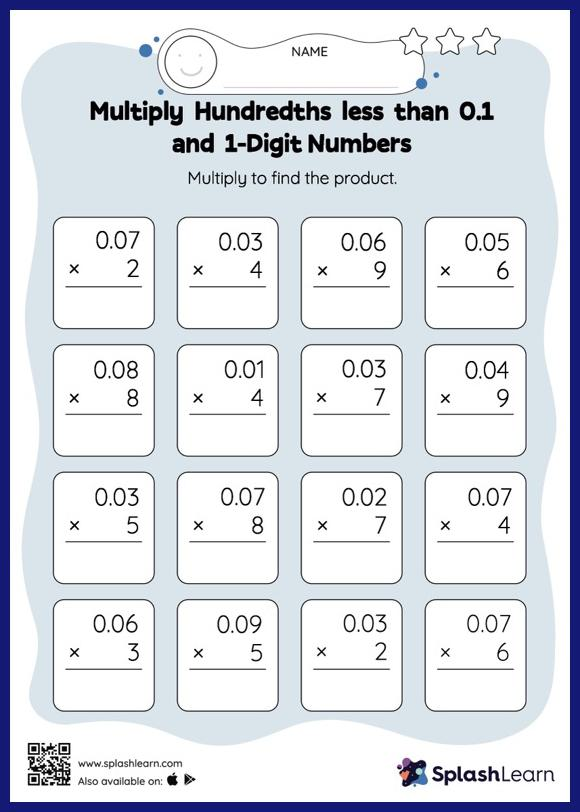# Multiply Hundredths less than 0.1 and 1-Digit Numbers: Vertical Multiplication Worksheet

Home > Multiply Hundredths less than 0.1 and 1-Digit Numbers: Vertical MultiplicationThis multiply hundredths less than 0.1 And 1-digit numbers worksheet consists of a set of questions on multiplication, which help students develop fluency. When multiplying a decimal by a one-digit number, students may ignore the decimal point during calculations and bring it back when writing the result. This multiply hundredths less than 0.1 And 1-digit numbers worksheet provides plenty of practice with this concept.